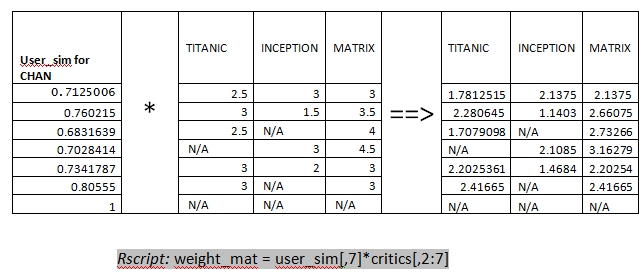# BigDataNews

A Data Science Central Community

Originally posted here

In our day to day life, we come across a large number of Recommendation engines like Facebook Recommendation Engine for Friends’ suggestions, and suggestions of similar Like Pages, Youtube recommendation engine suggesting videos similar to our previous searches/preferences. In today’s blog post I will explain how to build a basic recommender System.#### Types of Collaborative Filtering:

1. User based Collaborative Filtering
2. Item based Collaborative filtering

In this post will explain about User based Collaborative Filtering. This algorithm usually works by searching a large group of people and finding a smaller set with tastes similar to yours. It looks at other things they like and combines them to create a ranked list of suggestions.

#### Implementing User Based Collaborative Filtering:

This involves two steps:

1. Calculating Similarity Function
2. Recommend items to users based on user Similarity Score
Consider the below data sample of Movie critics and their movie rankings, the objective is to recommend the unrated movies based on similar users:

#### Step1- Calculate Similarity Score for CHAN:

Creating Similarity score for people helps us to identify similar people. We use Cosine based Similarity function to calculate the similarity between the users. Know more about cosine similarity here. In R we have a cosine function readily available:

user_sim = cosine(as.matrix(t(x)))

#### Step2- recommending Movies for CHAN:

For recommending movies for Chan using the above similarity matrix, we need to first fill the N/A where he has not rated. As first step, separate the non-rated movies by Chan and a weighted matrix is created by multiplying user similarity score (user_sim[,7]) with ratings given by other users.Next step is to sum up all the columns of the weight matrix, then divide by the sum of all the similarities for critics that reviewed that movie. The result calculation gives what the user might rate this movie, the results as below:The above explanation is written in the below R function:

rec_itm_for_user = function(userNo)
{ #calcualte column wise sum
col_sums= list()
rat_user = critics[userNo,2:7]
x=1
tot = list()
z=1
for(i in 1:ncol(rat_user)){
if(is.na(rat_user[1,i]))
{
col_sums[x] = sum(weight_mat[,i],na.rm=TRUE)
x=x+1
temp = as.data.frame(weight_mat[,i])
sum_temp=0
for(j in 1:nrow(temp))
{ if(!is.na(temp[j,1]))
{
sum_temp = sum_temp+user_sim[j,7]
}
}
tot[z] = sum_temp z=z+1
}
}
z=NULL
z=1
for(i in 1:ncol(rat_user)){
if(is.na(rat_user[1,i]))
{
rat_user[1,i] = col_sums[[z]]/tot[[z]] z=z+1
}
}
return(rat_user)
}

Calling the above function gives the below results:

rec_itm_for_user(7)
Titanic Batman Inception Superman.Returns spiderMan Matrix
2.811   4.5     2.355783           4            1    3.481427

Recommending movies for Chan will be in the order: Matrix (3.48), Titanic(2.81), Inception(2.35).

Views: 5523

Comment